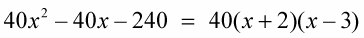##### Linear Algebra For Dummies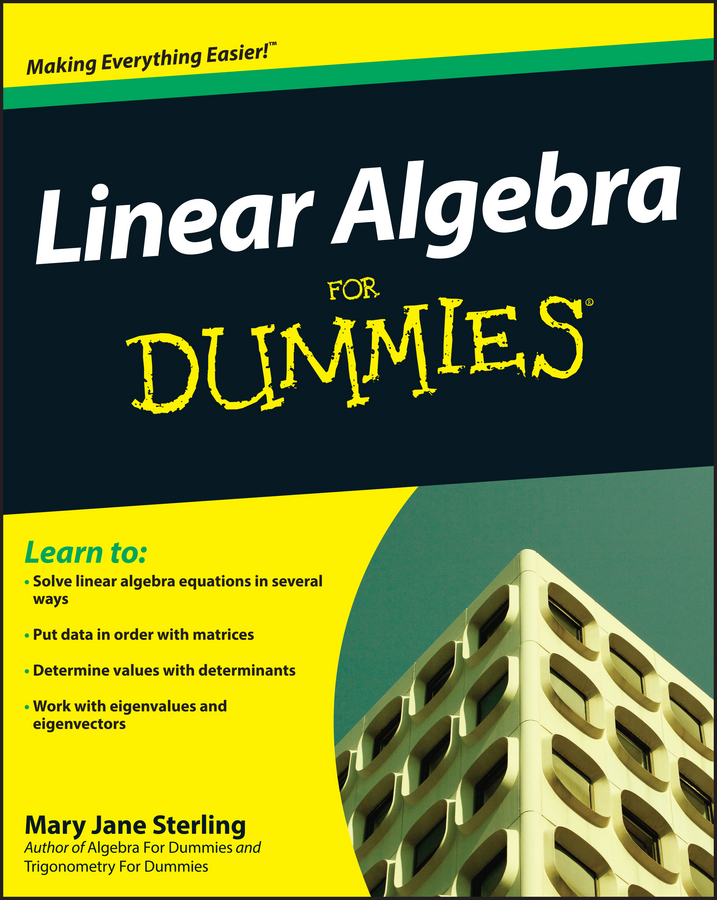Sometimes you factor expressions more than once — and with different factoring techniques. To determine if an expression needs to be factored more than once, just take another look at the expression after you first factor it to see if you need to factor it again.

Usually, you can get to the correct answer regardless of which factoring method — and whatever order — you choose. If you have to factor an expression more than once, however, the first step should always be an attempt to factor out the greatest common factor.

Example: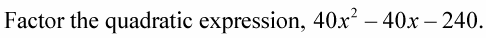1. Factor out the greatest common factor.

40 is the greatest common factor.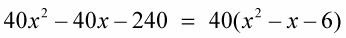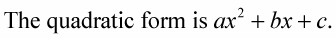1. Determine all the ways you can multiply two numbers to get a.

In this case, a is 1.

The 1 can be written only as 1 × 1.

2. Determine all the ways you can multiply two numbers to get c.

In this case, c is 6.

The 6 can be written as 1 × 6 or 2 × 3.

3. Look at the sign of c and your lists from Steps 1 and 2 to see if you want a sum or difference.

If c is positive, find a value from your Step 1 list and another from your Step 2 list such that the sum of their product and the product of the two remaining numbers in those steps results in b.

If c is negative, find a value from your Step 1 list and another from your Step 2 list such that the difference of their product and the product of two remaining numbers from those steps results in b.

In this case, c is negative, so you want the difference of the products to be 1.

4. Choose a product from Step 1 and a product from Step 2 that result in the correct sum or difference determined in Step 3.

You need a combination that results in the difference of 1.

Using 1 × 1 and 2 × 3, multiply (1)(2) to get 2, and multiply (1)(3) to get 3. The difference of these products equals 1.

5. Arrange your choices in the binomials so the results are those you want.

(1x 2)(1x 3)

6. Place the signs to give the desired results.

The middle term, x, is negative, so you want the 3x, the outer terms, to be negative.

(x + 2)(x – 3)

7. FOIL the two binomials to check your work.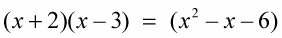3. Put the result of both factoring methods together.

Put back into the answer the 40 that you factored out at the beginning.